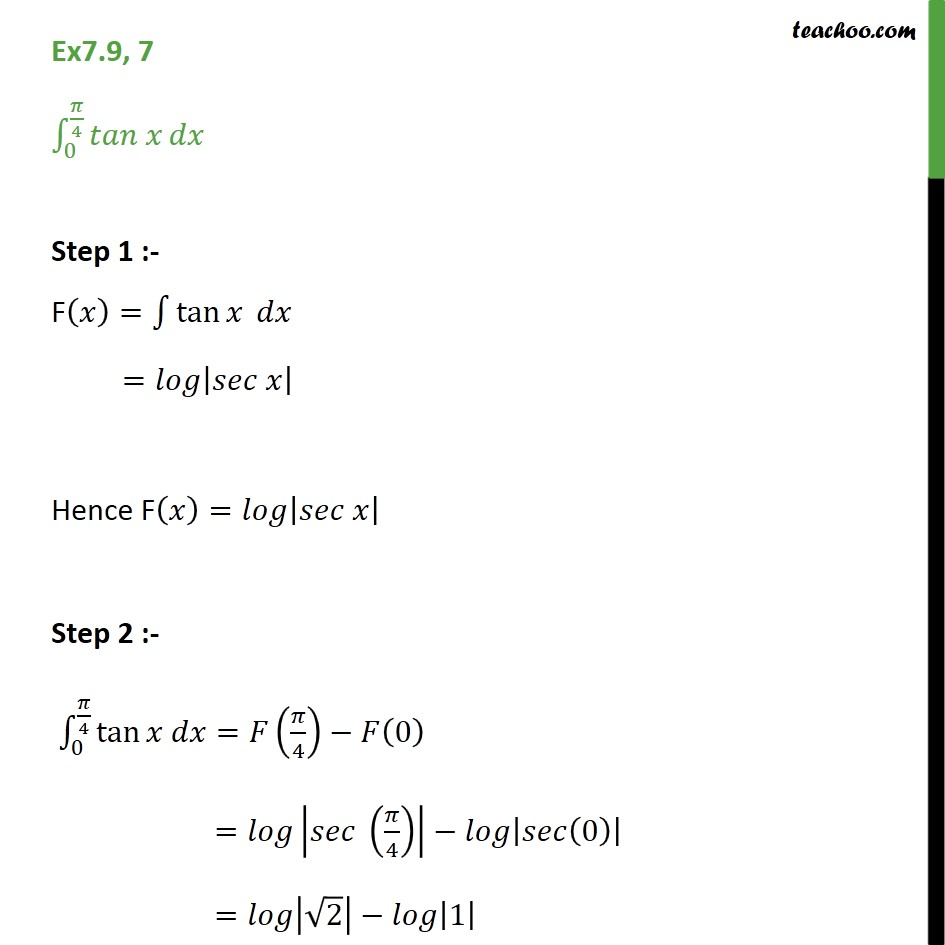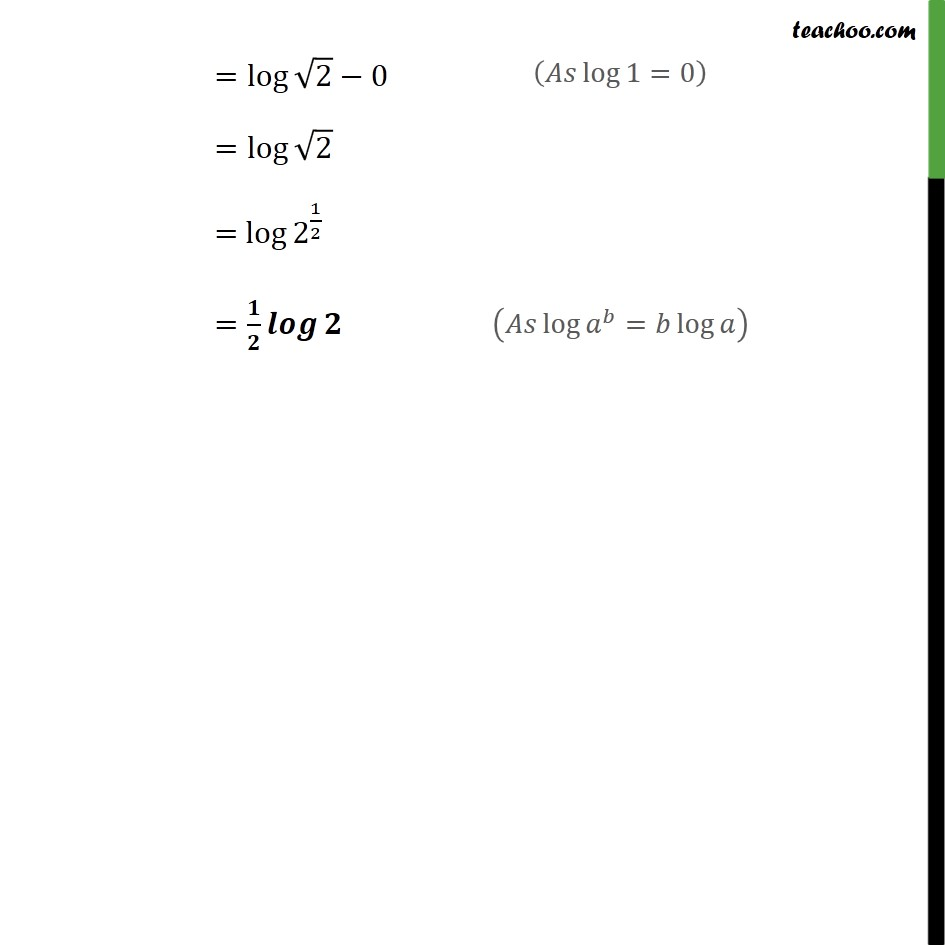Ex 7.9

Chapter 7 Class 12 Integrals
Serial order wiseGet live Maths 1-on-1 Classs - Class 6 to 12

### Transcript

Ex7.9, 7 0 4 Step 1 :- F = tan = Hence F = Step 2 :- 0 4 tan = 4 0 = 4 0 = 2 1 = log 2 0 = log 2 = log 2 1 2 =